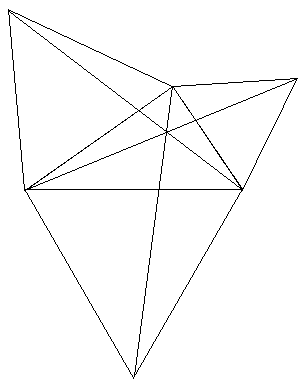# A problem due to FermatFermat posed the problem
Given a triangle, find the point where the sum of the distances to the vertices is a minimum.
Here is a solution
Given any triangle, construct an equilateral triangle on each side and join the vertices as shown.

These three lines meet at 60° in the so-called Fermat point.
(Actually, if the triangle has an angle ≥ 120° then this vertex gives the smallest sum.)